Technical Test SSC JE: Electrical Engineering (EE)- 6

# Technical Test SSC JE: Electrical Engineering (EE)- 6

Test Description

## 100 Questions MCQ Test Mock Test Series for SSC JE Electrical Engineering | Technical Test SSC JE: Electrical Engineering (EE)- 6

Technical Test SSC JE: Electrical Engineering (EE)- 6 for Electrical Engineering (EE) 2022 is part of Mock Test Series for SSC JE Electrical Engineering preparation. The Technical Test SSC JE: Electrical Engineering (EE)- 6 questions and answers have been prepared according to the Electrical Engineering (EE) exam syllabus.The Technical Test SSC JE: Electrical Engineering (EE)- 6 MCQs are made for Electrical Engineering (EE) 2022 Exam. Find important definitions, questions, notes, meanings, examples, exercises, MCQs and online tests for Technical Test SSC JE: Electrical Engineering (EE)- 6 below.
Solutions of Technical Test SSC JE: Electrical Engineering (EE)- 6 questions in English are available as part of our Mock Test Series for SSC JE Electrical Engineering for Electrical Engineering (EE) & Technical Test SSC JE: Electrical Engineering (EE)- 6 solutions in Hindi for Mock Test Series for SSC JE Electrical Engineering course. Download more important topics, notes, lectures and mock test series for Electrical Engineering (EE) Exam by signing up for free. Attempt Technical Test SSC JE: Electrical Engineering (EE)- 6 | 100 questions in 100 minutes | Mock test for Electrical Engineering (EE) preparation | Free important questions MCQ to study Mock Test Series for SSC JE Electrical Engineering for Electrical Engineering (EE) Exam | Download free PDF with solutions
 1 Crore+ students have signed up on EduRev. Have you?
Technical Test SSC JE: Electrical Engineering (EE)- 6 - Question 1

### In a transformer

Technical Test SSC JE: Electrical Engineering (EE)- 6 - Question 2

### One 20 V, 100 W bulb is connected in series with the primary of a 200 V, 10 kVA transformer. If its secondary is open circuited, then the bulb will have

Detailed Solution for Technical Test SSC JE: Electrical Engineering (EE)- 6 - Question 2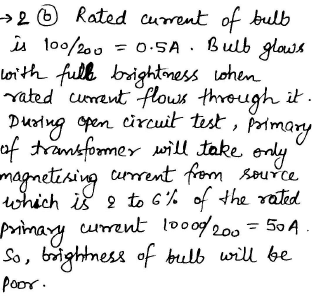Technical Test SSC JE: Electrical Engineering (EE)- 6 - Question 3

### A metal resistor has resistance of 10W at OºC and 11 ohms at 160ºC, the temperature coefficient is

Detailed Solution for Technical Test SSC JE: Electrical Engineering (EE)- 6 - Question 3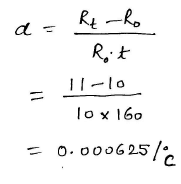Technical Test SSC JE: Electrical Engineering (EE)- 6 - Question 4

A 100 W, 200 V filament lamp has operating temperature of 2000ºC. The filament material has resistance temperature coefficient of 0.005 at OºC per ºC. The current taken by the lamp at the instant of switching with 200 V supply with filament temperature of 20ºC will be

Detailed Solution for Technical Test SSC JE: Electrical Engineering (EE)- 6 - Question 4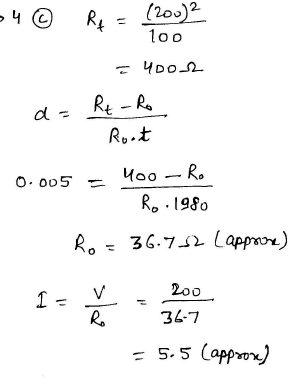Technical Test SSC JE: Electrical Engineering (EE)- 6 - Question 5

Which one of the following has the least value of form factor?

Detailed Solution for Technical Test SSC JE: Electrical Engineering (EE)- 6 - Question 5

In square wave, the rms value and average value are same (whatever peak magnitude presents in that square wave). So, the form factor is equal to one. But, in other kind of waveforms (for example, sine, triangle, rectangle, etc), the average value is less than the rms value. So, the value of form factor is greater than one. Hence, as compared to other waveforms, square wave has least value of form factor.

Technical Test SSC JE: Electrical Engineering (EE)- 6 - Question 6

Power factor of the magnetising component of a transformer is

Technical Test SSC JE: Electrical Engineering (EE)- 6 - Question 7

When a 100 mA alternating current with a frequency of 1 kHz flows in the primary of two coupled coils, the secondary voltage is 1V. The mutual inductance between the coils is

Detailed Solution for Technical Test SSC JE: Electrical Engineering (EE)- 6 - Question 7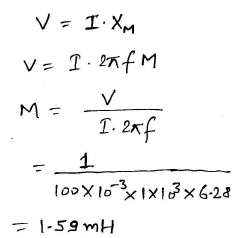Technical Test SSC JE: Electrical Engineering (EE)- 6 - Question 8

A coil with 1000 turns carrying a current of 8A produces a flux of 6×10–6 Wb. The inductance of the coil would be

Detailed Solution for Technical Test SSC JE: Electrical Engineering (EE)- 6 - Question 8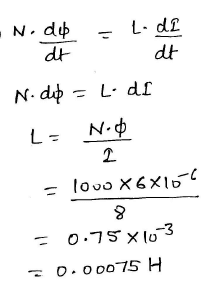Technical Test SSC JE: Electrical Engineering (EE)- 6 - Question 9

A shunt generator produces 450A at 230 V. The resistance of shunt field and armature are 50W and 0.025W respectively. The armature voltage drop will be

Detailed Solution for Technical Test SSC JE: Electrical Engineering (EE)- 6 - Question 9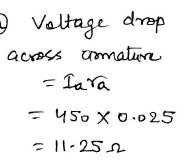Technical Test SSC JE: Electrical Engineering (EE)- 6 - Question 10

A 4-pole dynamo with wave wound armature has 51 slots containing 20 conductors. The voltage induced is 357 V and the speed is 8500 rpm. The flux per pole will be

Detailed Solution for Technical Test SSC JE: Electrical Engineering (EE)- 6 - Question 10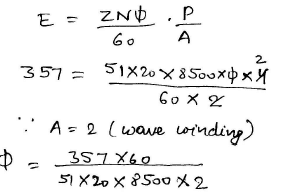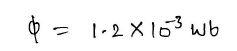Technical Test SSC JE: Electrical Engineering (EE)- 6 - Question 11

Series reactors are installed at strategic locations of power system to

Technical Test SSC JE: Electrical Engineering (EE)- 6 - Question 12

The inductance is introduced in the cables in the form of

Technical Test SSC JE: Electrical Engineering (EE)- 6 - Question 13

Load saturation characteristics of a dc generator gives relation between

Technical Test SSC JE: Electrical Engineering (EE)- 6 - Question 14

Equalizer rings are required in lap winding dc machine

Technical Test SSC JE: Electrical Engineering (EE)- 6 - Question 15

According to the fuse law, the current carrying capacity varies as

Detailed Solution for Technical Test SSC JE: Electrical Engineering (EE)- 6 - Question 15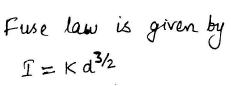Technical Test SSC JE: Electrical Engineering (EE)- 6 - Question 16

In electrodynamometer, ammeter, the deflection of the pointer is proportional to

Technical Test SSC JE: Electrical Engineering (EE)- 6 - Question 17

In which of the following transformers, is the secondary winding always kept closed ?

Technical Test SSC JE: Electrical Engineering (EE)- 6 - Question 18

Which of the following transducer requires a high input impedance preamplifier for proper measurements ?

Technical Test SSC JE: Electrical Engineering (EE)- 6 - Question 19

The shape of the curve between the flux density and ampere turns/m is correctly represented by which of the following figures ?

Technical Test SSC JE: Electrical Engineering (EE)- 6 - Question 20

Which loss in a dc generator significantly varies with the load current ?

Technical Test SSC JE: Electrical Engineering (EE)- 6 - Question 21

The ratio of starting torque to fully load torque is least in case of

Detailed Solution for Technical Test SSC JE: Electrical Engineering (EE)- 6 - Question 21

Explanation : From torque current characteristic, we get that torque at no load or starting torque is equal to zero. As load increases, speed decreases and armature current increases so as torque also increases linearly, if effect of armature reaction is neglected.

Technical Test SSC JE: Electrical Engineering (EE)- 6 - Question 22

A rheostat differs from potentiometer in the respect that

Technical Test SSC JE: Electrical Engineering (EE)- 6 - Question 23

The equivalent resistance at the points X1 and X2 in the circuit shown below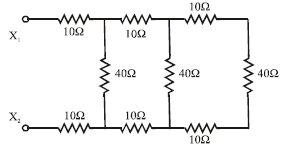Detailed Solution for Technical Test SSC JE: Electrical Engineering (EE)- 6 - Question 23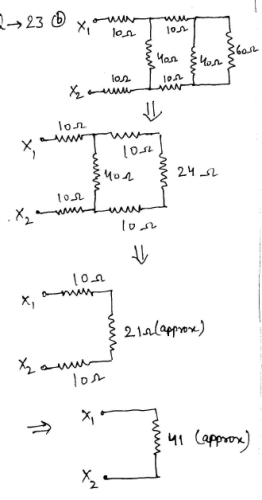Technical Test SSC JE: Electrical Engineering (EE)- 6 - Question 24

With the sole purpose of an alternating current is to produce heat, the selection of conductor is based on

Technical Test SSC JE: Electrical Engineering (EE)- 6 - Question 25

In case of delta connected circuit, when one resistor is open, power will be

Detailed Solution for Technical Test SSC JE: Electrical Engineering (EE)- 6 - Question 25

Explanation : Power consumed in case of balanced delta connection P = Vph2/Req. Req = 2R/3.

So, P = 3Vph2/2R.

If one resistor is removed from a 3 - phase delta connected circuit, then the circuit will be converted into single phase circuit having two resistors in series.

P’ = Vph2/Req. Req = 2R.

So, P’ = Vph2/2R.

P’ = P/3

Technical Test SSC JE: Electrical Engineering (EE)- 6 - Question 26

Permitivity is expressed in terms of

Technical Test SSC JE: Electrical Engineering (EE)- 6 - Question 27

The transition region in an open circuited p-n junction contains

Technical Test SSC JE: Electrical Engineering (EE)- 6 - Question 28

In an unbiased p-n junction, zero current implies that

Technical Test SSC JE: Electrical Engineering (EE)- 6 - Question 29

A null type of instruments as compared to a deflection type instrument has

Technical Test SSC JE: Electrical Engineering (EE)- 6 - Question 30

Accelerated again is done on coils for standard resistors in order to

Technical Test SSC JE: Electrical Engineering (EE)- 6 - Question 31

Technical Test SSC JE: Electrical Engineering (EE)- 6 - Question 32

Regenerative braking on shunt motors is used when

Technical Test SSC JE: Electrical Engineering (EE)- 6 - Question 33

Which of the following methods is most effective in finding out the no load losses in a large dc shunt motor ?

Technical Test SSC JE: Electrical Engineering (EE)- 6 - Question 34

Which domestic utility item has highest power rating ?

Technical Test SSC JE: Electrical Engineering (EE)- 6 - Question 35

Which equipment provides fluctuating loads ?

Technical Test SSC JE: Electrical Engineering (EE)- 6 - Question 36

In case of medium sized induction motor the power factor will be maximum at

Technical Test SSC JE: Electrical Engineering (EE)- 6 - Question 37

Efficiency is secondary consideration in case of

Technical Test SSC JE: Electrical Engineering (EE)- 6 - Question 38

In a transmission line following are the distributed constants

Technical Test SSC JE: Electrical Engineering (EE)- 6 - Question 39

In case the characteristic impedance of the line is equal to the load impedance

Technical Test SSC JE: Electrical Engineering (EE)- 6 - Question 40

Which type of insulators are used on 132KV transmission lines ?

Technical Test SSC JE: Electrical Engineering (EE)- 6 - Question 41

Under no load conditions, the current in a transmission line is due to

Technical Test SSC JE: Electrical Engineering (EE)- 6 - Question 42

A bus bar is rated by

Technical Test SSC JE: Electrical Engineering (EE)- 6 - Question 43

The function of breather in a transformer is

Technical Test SSC JE: Electrical Engineering (EE)- 6 - Question 44

Which loss in a transformer varies significantly with load ?

Technical Test SSC JE: Electrical Engineering (EE)- 6 - Question 45

Voltage remaining constant, if the frequency is increased then

Technical Test SSC JE: Electrical Engineering (EE)- 6 - Question 46

A parallel plate capacitor is filled with two dielectrics of E1 and E2 lengthwise equally. The
capacitance of the combination is

Detailed Solution for Technical Test SSC JE: Electrical Engineering (EE)- 6 - Question 46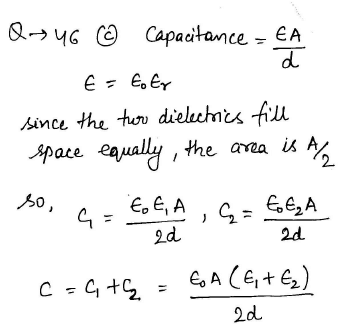Technical Test SSC JE: Electrical Engineering (EE)- 6 - Question 47

The supply voltage |V| in the diagram below is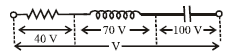Detailed Solution for Technical Test SSC JE: Electrical Engineering (EE)- 6 - Question 47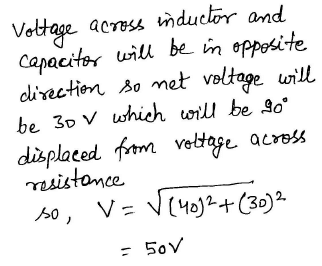Technical Test SSC JE: Electrical Engineering (EE)- 6 - Question 48

If the secondary of a 1:10 step up transformer is connected to the primary of a 1:5 step up transformer, the total transformation ratio will be

Technical Test SSC JE: Electrical Engineering (EE)- 6 - Question 49

Which of the following transformers is smallest ?

Technical Test SSC JE: Electrical Engineering (EE)- 6 - Question 50

When transistors are used in digital circuits they usually operate in the

Technical Test SSC JE: Electrical Engineering (EE)- 6 - Question 51

Three different Q points are shown on a dc load line. The upper Q point represents the

Technical Test SSC JE: Electrical Engineering (EE)- 6 - Question 52

Junction field effect transistor (JFET) contain how many diodes ?

Technical Test SSC JE: Electrical Engineering (EE)- 6 - Question 53

The ratio of available power from the dc component of a full-wave rectified sinusoid to the available power of the rectified sinusoid is

Detailed Solution for Technical Test SSC JE: Electrical Engineering (EE)- 6 - Question 53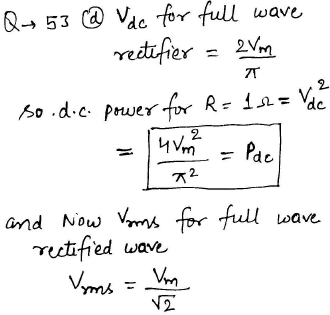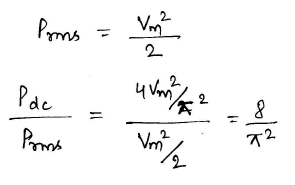Technical Test SSC JE: Electrical Engineering (EE)- 6 - Question 54

If the resistance in a series RC circuit is increased, the magnitude of the phase

Technical Test SSC JE: Electrical Engineering (EE)- 6 - Question 55

Two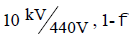transformer of ratings 600 kVA and 350 kVA are connected in parallel to share a load of 800 kVA. The reactance of the transformers, referred to the secondary sideare 0.0198W and 0.0304W respectively (resistance negligible). The load shared by the two transformers will be, respectively

Detailed Solution for Technical Test SSC JE: Electrical Engineering (EE)- 6 - Question 55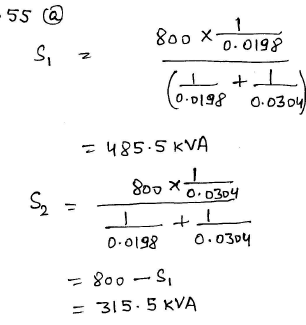Technical Test SSC JE: Electrical Engineering (EE)- 6 - Question 56

A separately exited dc motor has an armature resistance of 0.5W. It runs off a 250V dcsupply drawing an armature current of 20A at 1500 rpm. The torque developed for an armature current of 10A, for the same fieldcurrent, will be

Detailed Solution for Technical Test SSC JE: Electrical Engineering (EE)- 6 - Question 56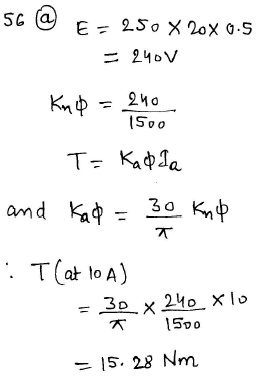Technical Test SSC JE: Electrical Engineering (EE)- 6 - Question 57

A generating station has a maximum demand of 50 MW, a load factor of 60%, a plant capacity factor of 45% and if the plant while running as per schedule, were fully loaded, running energy produced will be

Detailed Solution for Technical Test SSC JE: Electrical Engineering (EE)- 6 - Question 57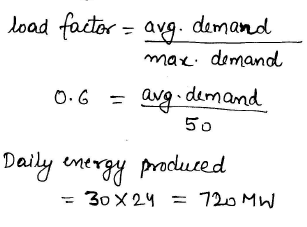Technical Test SSC JE: Electrical Engineering (EE)- 6 - Question 58

A distribution transformer usually is a

Technical Test SSC JE: Electrical Engineering (EE)- 6 - Question 59

Which of the following distribution system is the simplest and have lowest installation cost

Technical Test SSC JE: Electrical Engineering (EE)- 6 - Question 60

A random noise generator produces a signal

Technical Test SSC JE: Electrical Engineering (EE)- 6 - Question 61

A zener diode voltage regulator has load requirement of 12V and 2 Amp. The zener diode's minimum current requirement is 0-2A. The minimum voltage at input is 24V. What isthe maximum efficiency of circuit ?

Detailed Solution for Technical Test SSC JE: Electrical Engineering (EE)- 6 - Question 61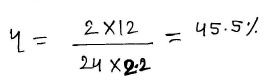Technical Test SSC JE: Electrical Engineering (EE)- 6 - Question 62

With the positive probe on an NPN base, an ohmmeter reading between the other transistor terminal should be

Technical Test SSC JE: Electrical Engineering (EE)- 6 - Question 63

The principle of operation of an LVDT is based on variation of

Technical Test SSC JE: Electrical Engineering (EE)- 6 - Question 64

Rectifier moving coil instruments respond to

Technical Test SSC JE: Electrical Engineering (EE)- 6 - Question 65

To the y-input of a CRO a signal defined by 10 sin 100t is applied. To the x-input, the signal 10 cos 100t is fed. The gain for both x-channel and y-channel is same. The screen shows

Detailed Solution for Technical Test SSC JE: Electrical Engineering (EE)- 6 - Question 65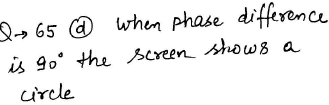Technical Test SSC JE: Electrical Engineering (EE)- 6 - Question 66

A short circuit test on a transformer gives

Technical Test SSC JE: Electrical Engineering (EE)- 6 - Question 67

Which of the following connection of transformer will give the highest secondary voltage ?

Technical Test SSC JE: Electrical Engineering (EE)- 6 - Question 68

For an alternator when the power factor of the load is unity

Technical Test SSC JE: Electrical Engineering (EE)- 6 - Question 69

The advantage of salient poles in an alternator is

Technical Test SSC JE: Electrical Engineering (EE)- 6 - Question 70

In a 440V system, in order to obtain the minimum cost and maximum benefits, the capacitor should be installed

Technical Test SSC JE: Electrical Engineering (EE)- 6 - Question 71

Which of the following relations between apparent power (kVA), true power (kW) and reactive power kVAR is true ?

Technical Test SSC JE: Electrical Engineering (EE)- 6 - Question 72

Power distribution by cable is generally adopted for line length

Technical Test SSC JE: Electrical Engineering (EE)- 6 - Question 73

Supplier's fuse, which is provided in domestic wiring system is

Technical Test SSC JE: Electrical Engineering (EE)- 6 - Question 74

A supply voltage of 230V, 50 Hz is fed to a residential welding. Write down its equation for instantaneous value

Technical Test SSC JE: Electrical Engineering (EE)- 6 - Question 75

A transistor has a Bdc of 250 and a base current, IB of 20 mA. The collector current IC, equals

Detailed Solution for Technical Test SSC JE: Electrical Engineering (EE)- 6 - Question 75

A collector current is the current, directly passing in the transistor through a collector.

The formula to determine the collector current is -

beta = Ic/Ib

Where Ic = 20 mA,

Therefore Ib -

Ic = Beta × Ib

Ic = 250 × 20/1000000

Ic = 5000/1000000

Ic = 5

Answer: The collector current is 5mA

Technical Test SSC JE: Electrical Engineering (EE)- 6 - Question 76

In a CE configuration, an emitter resistor is used for

Technical Test SSC JE: Electrical Engineering (EE)- 6 - Question 77

A diode can be used as a frequency multiplier because of its

Technical Test SSC JE: Electrical Engineering (EE)- 6 - Question 78

Which of the following is not characteristics of an oscillator ?

Technical Test SSC JE: Electrical Engineering (EE)- 6 - Question 79

You can find the zener diode in

Technical Test SSC JE: Electrical Engineering (EE)- 6 - Question 80

A series resonant circuit has an inductive reactance of 1000W, a capacitive reactance of 1000W and a resistance of 0.1W. If the resonant frequency is 10 MHz, then the bandwidth of the circuit will be

Detailed Solution for Technical Test SSC JE: Electrical Engineering (EE)- 6 - Question 80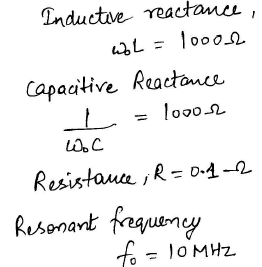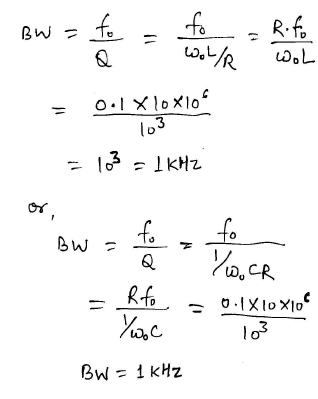Technical Test SSC JE: Electrical Engineering (EE)- 6 - Question 81

The circuit shown in figure-I is replaced by that in figure-II. If current 'I' remains the same than Ro will be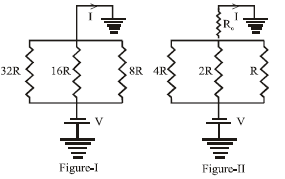Detailed Solution for Technical Test SSC JE: Electrical Engineering (EE)- 6 - Question 81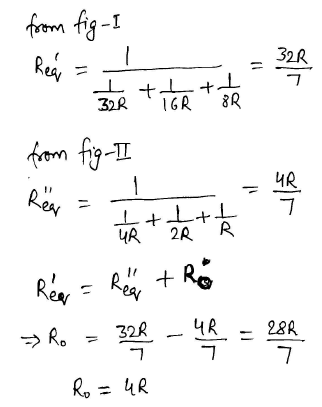Technical Test SSC JE: Electrical Engineering (EE)- 6 - Question 82

When the resistor voltage in a series RL circuit becomes less than the inductor voltage, the phase angle :

Detailed Solution for Technical Test SSC JE: Electrical Engineering (EE)- 6 - Question 82

As the inductor voltage decreases, the voltage across the resistor increases and therefore the current also increases. Ultimately, the inductor voltage is zero, all of the voltage source output is across the resistor, and the current is at its maximum value.When the resistor voltage in a series RL circuit becomes less than the inductor voltage, the phase angle increases.

Technical Test SSC JE: Electrical Engineering (EE)- 6 - Question 83

Diversity factor is equal to

Technical Test SSC JE: Electrical Engineering (EE)- 6 - Question 84

The starting capacitor of a single phase motor is

Technical Test SSC JE: Electrical Engineering (EE)- 6 - Question 85

The short coming of repulsion motor is

Technical Test SSC JE: Electrical Engineering (EE)- 6 - Question 86

A belt-driven cumulatively-compounded dc generator is delivering power to the dc mains. If the belt snaps then the machine will run as a

Detailed Solution for Technical Test SSC JE: Electrical Engineering (EE)- 6 - Question 86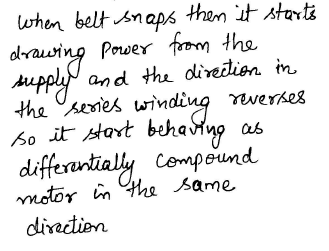Technical Test SSC JE: Electrical Engineering (EE)- 6 - Question 87

In a loaded dc generator, if the brushes are given a shift from the interpolar axis in the direction of rotation, then the commutation will

Detailed Solution for Technical Test SSC JE: Electrical Engineering (EE)- 6 - Question 87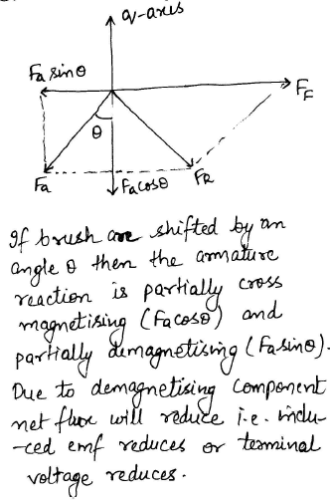Technical Test SSC JE: Electrical Engineering (EE)- 6 - Question 88

i1 in the circuit is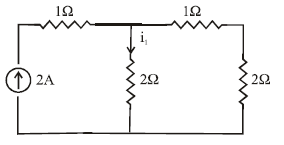Detailed Solution for Technical Test SSC JE: Electrical Engineering (EE)- 6 - Question 88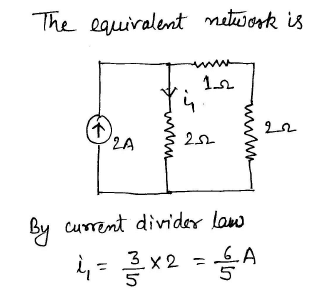Technical Test SSC JE: Electrical Engineering (EE)- 6 - Question 89

In a single phase power factor meter, the controlling torque is

Technical Test SSC JE: Electrical Engineering (EE)- 6 - Question 90

In calibration of a dynamometer wattmeter by potentiometer, phantom loading arrangement is used because

Technical Test SSC JE: Electrical Engineering (EE)- 6 - Question 91

The illumination level in houses is in the range

Technical Test SSC JE: Electrical Engineering (EE)- 6 - Question 92

One lumen per square metre is the same as

Technical Test SSC JE: Electrical Engineering (EE)- 6 - Question 93

For the power factor correction of welding transformer, a capacitor is usually connected on

Technical Test SSC JE: Electrical Engineering (EE)- 6 - Question 94

The arc has

Technical Test SSC JE: Electrical Engineering (EE)- 6 - Question 95

The efficiency of a welding motor generator is usually in the range of

Technical Test SSC JE: Electrical Engineering (EE)- 6 - Question 96

Skewing of rotor slots helps in

Technical Test SSC JE: Electrical Engineering (EE)- 6 - Question 97

In a constant power type load

Technical Test SSC JE: Electrical Engineering (EE)- 6 - Question 98

If a cable is to be designed for use on 1000 kV which insulation would you prefer ?

Technical Test SSC JE: Electrical Engineering (EE)- 6 - Question 99

In a CRO, the focusing anode is located

Technical Test SSC JE: Electrical Engineering (EE)- 6 - Question 100

The mutual inductance between two coils having self inductances 3 henry and 12 henry and coupling coefficient 0.85 is

Detailed Solution for Technical Test SSC JE: Electrical Engineering (EE)- 6 - Question 100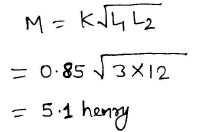## Mock Test Series for SSC JE Electrical Engineering

2 videos|1 docs|55 tests
 Use Code STAYHOME200 and get INR 200 additional OFF Use Coupon Code
Information about Technical Test SSC JE: Electrical Engineering (EE)- 6 Page
In this test you can find the Exam questions for Technical Test SSC JE: Electrical Engineering (EE)- 6 solved & explained in the simplest way possible. Besides giving Questions and answers for Technical Test SSC JE: Electrical Engineering (EE)- 6, EduRev gives you an ample number of Online tests for practice

## Mock Test Series for SSC JE Electrical Engineering

2 videos|1 docs|55 tests